# MCQ Questions for Class 7 Maths Chapter 5 Lines and Angles with Answers

We have compiled the NCERT MCQ Questions for Class 7 Maths Chapter 5 Lines and Angles with Answers Pdf free download covering the entire syllabus. Practice MCQ Questions for Class 7 Maths with Answers on a daily basis and score well in exams. Refer to the Lines and Angles Class 7 MCQs Questions with Answers here along with a detailed explanation.

## Lines and Angles Class 7 MCQs Questions with Answers

Choose the correct option.

Question 1.
The measure of the complement of the angle 40° is
(a) 15°
(b) 40°
(c) 50°
(d) 140°

Question 2.
When the sum of the measures of two angles is 90°, the angles are called
(b) complementary angles
(c) vertically opposite angles
(d) supplementary angles

Question 3.
Which of the following pairs can form a linear pair?
(a) Pair of complementary angles
(b) Pair of supplementary angles
(d) Pair of vertically opposite angles

Answer: (b) Pair of supplementary angles

Question 4.
If a transversal intersects two parallel lines then the interior angles on the same side of the transversal are
(a) vertically opposite angles
(b) supplementary angles
(c) complementary angles
(d) alternate angles

Question 5.
Which pair of the following angles are complementary?Question 6.
Which of the following is false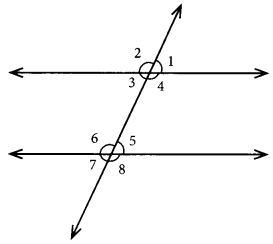(a) ∠1 = ∠2
(b) ∠2 = ∠4
(c) ∠2 + ∠3 = 180°
(d) ∠3 + ∠4 = 180°

Question 7.
Which angle is complementary to ∠CBD?(a) ∠FBC
(b) ∠EBF
(c) ∠ABC
(d) ∠DBE

Question 8.
In the given figure, the value of a is(a) 35°
(b) 45°
(c) 55°
(d) 110°

Question 9.
Find the value of x in the following figure if l || m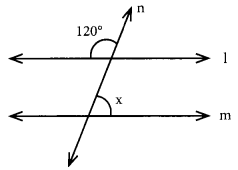(a) 30°
(b) 50°
(c) 60°
(d) 90°

Question 10.
In the given figure l || m. Find the measure of ‘x’.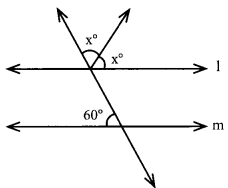(a) 120°
(b) 60°
(c) 45°
(d) 30°

Question 11.
The sum of two complementary angles is:
(a) 90°
(b) 180°
(c) 360°
(d) Any angle between 180° and 360°

Question 12.
The sum of two supplementary angles is:
(a) 90°
(b) 180°
(c) 360°
(d) Any angle between 180° and 360°

Look at the following figures and fill in the blanks

Question 1.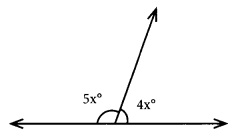x° = ………………..

Question 2.x° = ………………..

Question 3.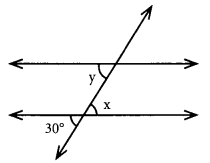∠x = ∠y = ……………..

Question 4.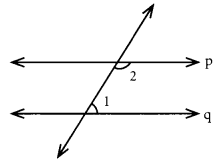If p and q are parallel lines then ∠1 + ∠2 = ……………..

Question 5.
Two angles are said to be …………………. if the sum of their measures is 90°.

Question 6.
Two angles are said to be …………………. if the sum of their measures is 180°.

Question 7.
The complement of 15° is ………………….

Question 8.
The …………………. of 10° is 170°.

Question 9.
The sum of two angles in a linear pair is ………………….

Question 10.
Two adjacent angles have a common …………………. and a common vertex.

Question 11.
Two …………………. adjacent angles form a linear pair.

Question 12.
If the sum of the measures of two angles is 90°, then each one of them is the …………………. of the other.

Question 13.
Parallel lines are always …………………. [equal/equidistant]

Question 14.
If two parallel lines are intersected by a transversal, then a pair of alternate angles are …………………. [equal/complementary]

Match the following